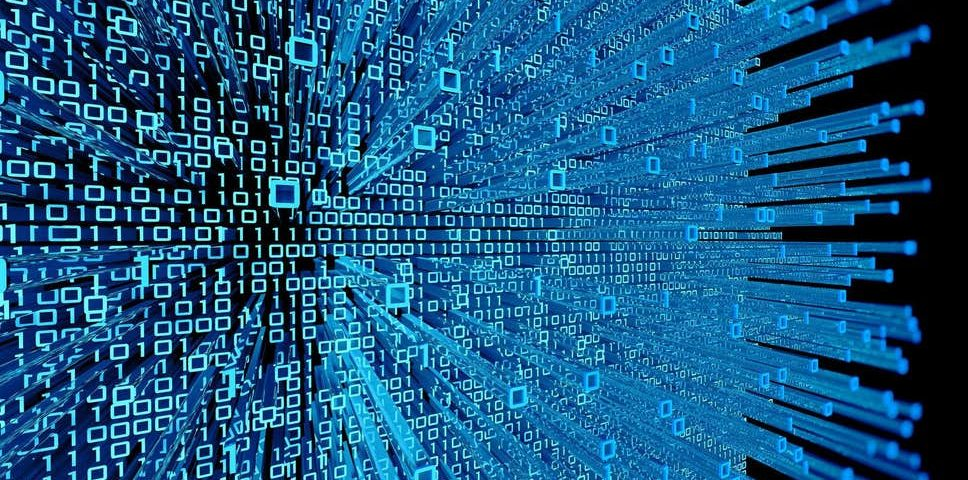# Quantum mechanics and quantum computing: Some philosophy and light mathematicsThe only way to really understand quantum mechanics (and quantum computing,  for which this knowledge is essential) is through mathematics, and this post is a very light introduction to the mathematics of quantum operations (and their interface with some philosophical issues). I have done it as a PDF as there is a fair amount of equations and other mathematical symbols in it, and I don't have time to set the latex syntax for getting the equations into Wordpress at the moment, as this is something of a coding operation in itself. Here is the PDF:

Quantum mechanics and quantum computation (PDF)

The first half is largely derived from Richard Feynman's wonderful textbook of quantum mechanics for physics undergraduates (Feynman lectures vol. 3) though the mathematics is straightforward, and the second is from a variety of IBM and Microsoft quantum computing resources.